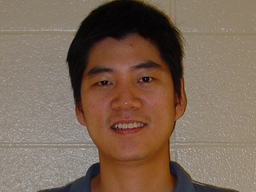← Back to Event List

# Doctoral Dissertation Defense: Sungwoo Choi

Location

Sondheim Hall : 209

Date & Time

May 7, 2014, 10:00 am12:00 pm

Description

Title:   Classification using the ROC curve analysis and testing non-equivalence

Abstract: The dissertation consists of two different topics. The first topic is to develop the methodologies in optimizing the ROC (Receiver Operating Characteristic) curve with variable selection based on group sparsity. An ROC curve is a popular tool in the classification of two populations. The nonparametric additive model is used to construct a classifier which is estimated by maximizing the U-statistic type of empirical AUC (Area Under Curve). In particular, the sparsity situation is considered in the sense that only a small number of variables is significant in the classification, so it is demanded that lots of noisy variables will be removed. Some theoretical result on the necessity of variable selection under the sparsity condition is provided since the AUC of the classifier from maximization of empirical AUC is not guaranteed to be optimal. To select significant variables in the classification, the grouped lasso which has been widely used when groups of parameters need to be either selected or discarded simultaneously is used. In addition, the performance of the proposed method is evaluated by numerical studies including simulation and real data examples compared with other existing approaches. The second topic is developing the hypothesis test for non-equivalence. We consider the problem of testing the non-equivalence of several independent normal population means. Testing homogeneity of several population means usually refers to testing the exact equality of population means. Instead of determining the exact homogeneity or equality, one may consider more flexible homogeneity which allows a predetermined level of difference. This problem is known as testing the non-homogeneity of populations. We propose the plug-in tests for three different measures of variability: the sum of the absolute deviations, the maximum of the absolute deviations, and the range for testing non-equivalence. For each test, the least favorable configuration (LFC) to ensure the maximum rejection probability under the null hypothesis is investigated. Furthermore, we demonstrate the numerical studies based on both simulations and real data to evaluate the plug-in tests and compare our proposed tests with other possible tests.
Tags: